Function Repository Resource:

Compute the adjacency tensor of an arbitrary hypergraph

Contributed by: Jonathan Gorard
 ResourceFunction["AdjacencyTensor"][h] gives the vertex adjacency tensor of the (ordered or orderless) hypergraph h.

## Details and Options

An adjacency tensor is a generalization of the concept of an adjacency matrix from graphs to hypergraphs, in which hyperedges may be of arbitrary arity.
The rank of the adjacency tensor is equal to the arity of the hyperedges in the hypergraph.
The adjacency tensor for a hypergraph will have dimensions n×n××n, where n is the number of vertices.
ResourceFunction["AdjacencyTensor"] returns a SparseArray object, which can be converted into ordinary nested lists using Normal.
Each entry aij…k of the adjacency tensor represents the number of hyperedges of the form {vi,vj,,vk}.
The diagonal entries aii…i count the number of self-loops for each vertex vi.
 "OrderedHyperedges" False whether to treat hyperedges as being ordered (directed)
When all hyperedges are of arity 2, the output of ResourceFunction["AdjacencyTensor"] is identical to that of AdjacencyMatrix.

## Examples

### Basic Examples (3)

The adjacency tensor of an orderless hypergraph, with hyperedges of arity 3:

 In:=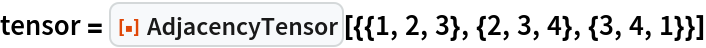Out=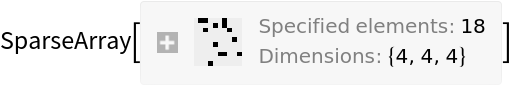In:=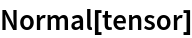Out=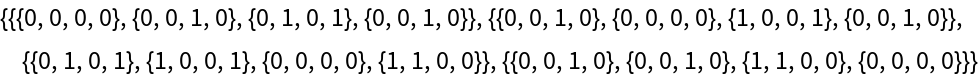The adjacency tensor of an ordered hypergraph, with hyperedges of arity 3:

 In:=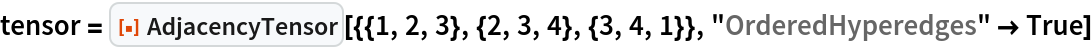Out=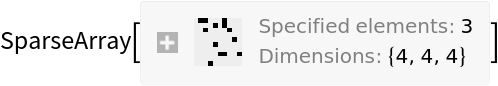In:=Out=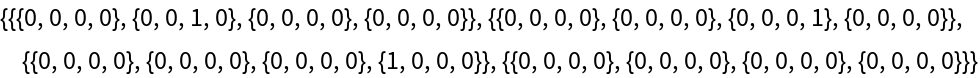The adjacency tensor of an orderless hypergraph, with hyperedges of arity 5:

 In:=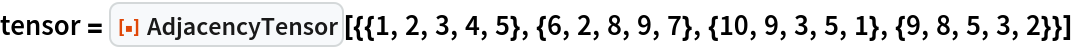Out=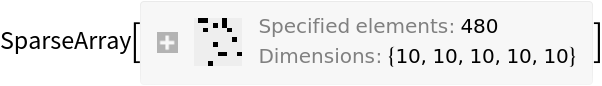### Scope (6)

AdjacencyTensor supports multihypergraphs, in which case the tensor entries represent hyperedge multiplicities:

 In:=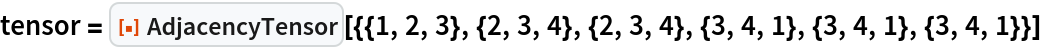Out=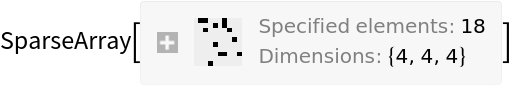In:=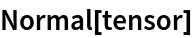Out=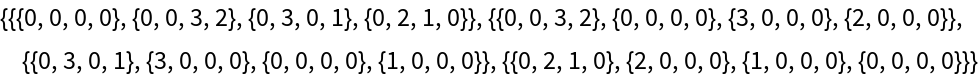When the arity of hyperedges is equal to 2, the output of AdjacencyTensor is identical to the output of AdjacencyMatrix:

 In:=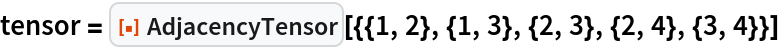Out=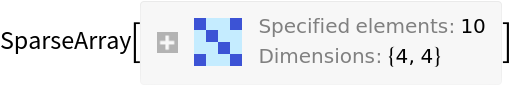In:=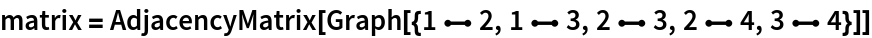Out=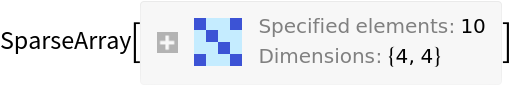In:=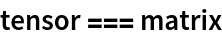Out=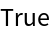The adjacency tensor of an orderless hypergraph is always symmetric across all indices:

 In:=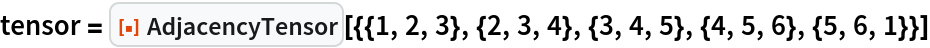Out=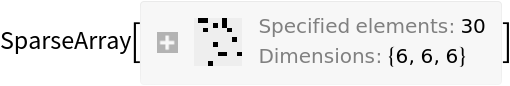In:=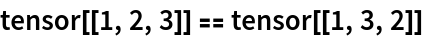Out=The adjacency tensor of an ordered hypergraph is not necessarily symmetric across all indices:

 In:=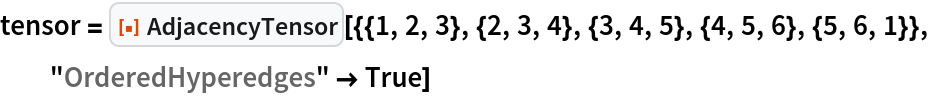Out=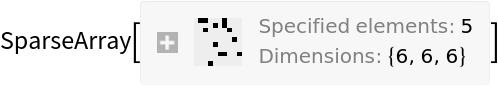In:=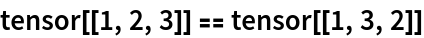Out=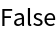The adjacency tensor of a hypergraph with self-loops has diagonal entries:

 In:=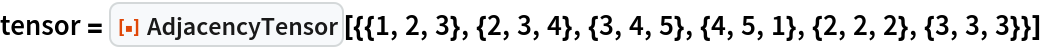Out=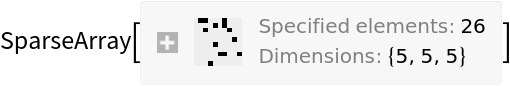In:=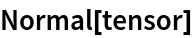Out=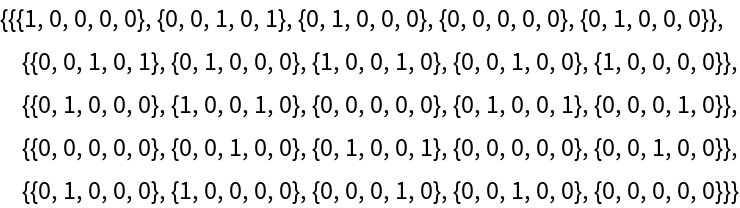In:=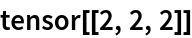Out=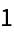Hyperedges can be of arbitrary arity:

 In:=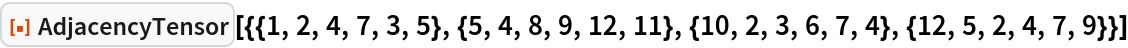Out=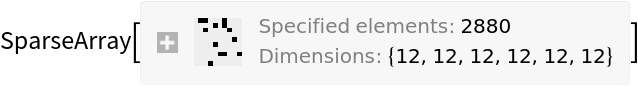### Options (2)

By default, all hyperedges are treated as orderless (i.e. undirected):

 In:=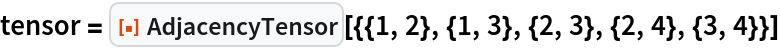Out=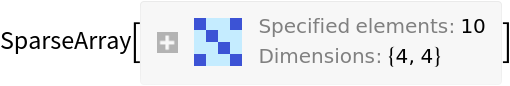In:=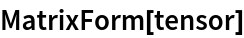Out=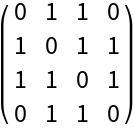Use "OrderedHyperedges"True to treat hyperedges as ordered (i.e. directed):

 In:=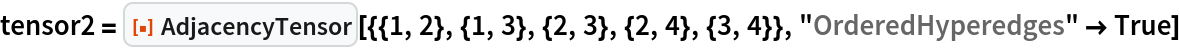Out=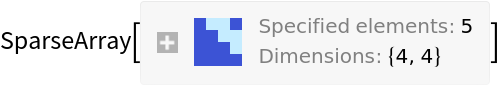In:=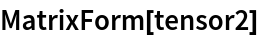Out=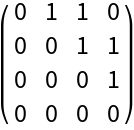Jonathan Gorard

## Version History

• 1.0.0 – 17 March 2021